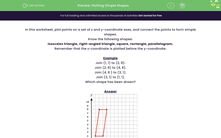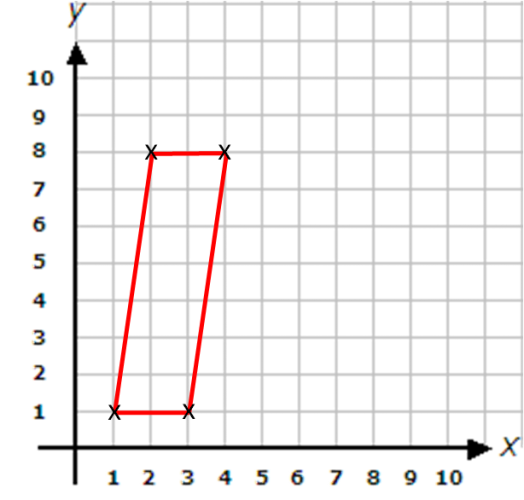# Plotting Simple Shapes

In this worksheet, students must plot points on a set of xy-coordinate axes and join certain points to form simple 2D shapes.Key stage:  KS 2

Curriculum topic:   Geometry: Position and Direction

Curriculum subtopic:   Plot Points and Draw Sides

Popular topics:   Geometry worksheets

Difficulty level:#### Worksheet Overview

In this worksheet, plot points on a set of x and y-coordinate axes, and connect the points to form simple shapes.

Know the following shapes:

Isosceles triangle, right-angled triangle, square, rectangle, parallelogram.

Remember that the x-coordinate is plotted before the y-coordinate.

Example

Join (1, 1) to (2, 8).

Join (2, 8) to (4, 8).

Join (4, 8 ) to (3, 1).

Join (3, 1) to (1, 1).

Which shape has been drawn?We have drawn a  parallelogram.

### What is EdPlace?

We're your National Curriculum aligned online education content provider helping each child succeed in English, maths and science from year 1 to GCSE. With an EdPlace account you’ll be able to track and measure progress, helping each child achieve their best. We build confidence and attainment by personalising each child’s learning at a level that suits them.

Get started